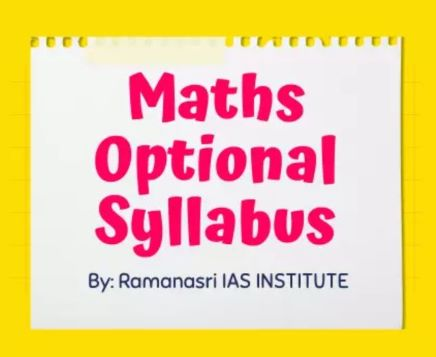Maths Optional Syllabus complete details.
Ramanasri UPSC Maths Optional Coaching providing complete details of Mains IAS, UPSC, IFoS, CSE-Civil Services Exams and State PCs Mains like BPSC, HPSC, JKPSC, UPPSC, WBCS/WBPSC, OPSC Maths Optional Syllabus.### Let's See Our Latest Details

:: UPPSC Maths Optional Syllabus PAPER - I ::

1. Linear Algebra and Matrix: Vector spaces, Sub Spaces, basis and dimensions, Quotient. space, co-ordinates, linear transformation, rank and nullity of a linear transformation, matrix representation of linear transformation, linear functionals, dual space, transpose of a linear transformation, characteristic values, annihilating polynomials, Cayley-Hamilton theorem, Inner product spaces, Cauchy-Schwarz inequality, Orthogonal vectors, orthogonal complements, orthonormal sets and bases, Bessel's inequality of finite dimensional spaces, Gram-Schmidt orthogonalisation process. Rank of Matrix, Echelon form, Equivalence, congruence and similarity, Reduction to canonical form, orthogonal, symmetrical, skew-symmetrical, Hermitian and skew-Hermitian matrices, their eigen values, orthogonal and unitary reduction of quadratic and Hermitian form, Positive definite quadratic forms, simultaneous reduction.

2. Calculus: Limits, continuity, differentiability, mean value theorems, Taylor's theorem, indeterminate forms, maxima and minima, tangent and normal, Asymptotes, curvature, envelope and evolute, curve tracing, continuity and differentiability of function of several variables Interchangeability of partial derivatives, Implicit functions theorem, double and triple integrals. (techniques only), application of Beta and Gamma functions, areas, surface and volumes, centre of gravity.

3. Analytical Geometry of two and three dimensions: General equation of second degree, system of conics, confocal conics, polar equation of conics and its properties. Three dimensional co-ordinates, plane, straight line, sphere, cone and cylinder. Central conicoids, paraboloids, plane section of conicoids, generating lines, confocal conicoids.

4. Ordinary differential equations: Order and Degree of a differential equation, linear, and exact differential equations of first order and first degree, equations of first order but not of first degree, Singular solutions, Orthogonal trajectories, Higher order linear equations with constant coefficients, Complementary functions and particular integrals.

Second order linear differential equations with variable coefficients: use of known solution to find another, normal form, method of undetermined coefficients method of variation of parameters.

5. Vector and Tensor Analysis: Vector Algebra, Differentiation and integration of vector function of a scalar variable gradient, divergence and curl in cartesian, cylindrical and spherical coordinates and their physical interpretation, Higher order derivates, vector identities and, vector equations, Green's, Gauss and Stoke's theorems

:: UPPSC Maths Optional Syllabus PAPER - II ::

1. Algebra: Groups, Cyclic groups, subgroups, Cosets of a subgroup, Lagrange's theorem, Normal subgroups, Homomorphism of groups, Factor groups, basic Isomorphism theorems, Permutation groups, Cayley's theorem.

Rings, Subrings, Ideals, Integral domains, Fields of quotients of an integral domain, Euclidean domains, Principal ideal domains, Polynomial rings over a field, Unique factorization domains.

2. Real Analysis: Metric spaces and their topology with special reference to sequence, Convergent sequence, Cauchy sequences, Cauchy's criterion of convergence, infinite series and their convergence, nth term test, series of positive terms, Ratio and root tests, limit comparison tests, logarithmic ratio test, condensation test, Absolute and conditional convergence of general series in R, Abel's Dirichlet's theorems. Uniform convergence of sequences and series of functions over an interval, Weierstrass M-test, Abel's and Dirichlet's tests, continuity of limit function. Term by term integrability and differentiability.

Riemann's theory of integration for bounded functions, integrability of continuous functions. Fundamental theorem of calculus. Improper integrals and conditions for their existence, tests.

3. Complex Analysis: Analytic functions, Cauchy-Riemann equations, Cauchy's theorem, Cauchy's integral formula, Power series representation of an analytic function. Taylor's series. Laurent's series, Classification of singularities, Cauchy's Residue theorem, Contour integration.

4. Partial Differential Equations: Formation of partial differential equations. Integrals of partial differential equations of first order, Solutions of quasi linear partial differential equations of first order, Charpit's method for non-linear partial differential equations of first order, Linear Partial differential equations of the second order with constant coefficients and their canonical forms, Equation of vibrating string. Heat equation. Laplace equation and their solutions.

5. Mechanics: Generalized co-ordinates, generalized velocities, Holonomic and nonholonomic systems, D'Alembert's principle and Lagrange's equations of motion for holonomic systems in a conservative field, generalized momenta, Hamilton's equations. Moments and products of inertia, Principal axes, Moment of inertia about a line with direction cosines (l,m,n), Momental ellipsoid, Motion of rigid bodies in two dimensions.

6. Hydrodynamics: Equation of continuity, Velocity Potential, Stream lines, Path Lines, Momentum and energy.  Inviscid flow theory: Euler's and Bernoulli's equations of motion. Two-dimensional fluid motion, Complex potential, Momentum and energy, Sources and Sinks, Doublets and their images with respect line and circle.

7. Numerical Analysis: Solution of algebraic and transcendental equations of one variable by bisection, Regula-Falsi and Newton-Raphson methods and order of their convergence. Interpolation (Newton's and Lagrange's) and Numerical differentiation formula with error terms. Numerical Integration: Trapezoidal and Simpson's rules. Numerical solutions of Ordinary differential Equations: Euler's method, Runge-Kutta method.

About UPPSC Maths Optional Syllabus in Hindi coming soon...!!!